﻿ Effect of Pretwist on Free Vibration Characteristics Determination of Metallic Curved Blade Replaced with Composite Material

### Effect of Pretwist on Free Vibration Characteristics Determination of Metallic Curved Blade Replaced...

M.L. Pavan Kishore, R.K. BeheraOPEN ACCESSPEER-REVIEWED

## Effect of Pretwist on Free Vibration Characteristics Determination of Metallic Curved Blade Replaced with Composite Material

M.L. Pavan Kishore1,, R.K. Behera1

1Department of Mechanical engineering, National Institute of Technology, Rourkela, India

### Abstract

This paper addresses the effects of a pretwist on the behavior of curved blade. The blade is considered to be clamped at one end on the axis of rotation. The vibration analysis of pre-twisted blade is performed by using finite element method based solver Ansys. The effects of pretwist on the natural frequencies and mode shapes of metallic curved blade replaced with composite are investigated. The methodology used to determine the Eigen frequency characteristics are determined and the results emphasizing the influence played by the ply angle, pretwist and stacking sequence on the free vibration characteristics are presented and pertinent conclusions are outlined.

1234
Prev Next

### Cite this article:

• Kishore, M.L. Pavan, and R.K. Behera. "Effect of Pretwist on Free Vibration Characteristics Determination of Metallic Curved Blade Replaced with Composite Material." Journal of Ocean Research 2.1 (2014): 11-16.
• Kishore, M. P. , & Behera, R. (2014). Effect of Pretwist on Free Vibration Characteristics Determination of Metallic Curved Blade Replaced with Composite Material. Journal of Ocean Research, 2(1), 11-16.
• Kishore, M.L. Pavan, and R.K. Behera. "Effect of Pretwist on Free Vibration Characteristics Determination of Metallic Curved Blade Replaced with Composite Material." Journal of Ocean Research 2, no. 1 (2014): 11-16.

 Import into BibTeX Import into EndNote Import into RefMan Import into RefWorks

### 1. Introduction

Rotating components have the shape of pre twisted blades find its applications commonly in several types of engineering & technology fields such as turbines, marine propulsion, wind mill blades, aircraft wings, turbo machines and aircraft rotary wings. The design of such rotating components includes determination of its modal characteristics which are to be determined accurately. Since the normal modes of motion and associated natural frequencies of vibration of blades depends on the magnitude of pretwist angle. The main objective in this paper is to study the effects of pretwist on the natural frequencies of curved blade. The pretwist angles ranging between 0 & π/2 are considered. A modeling method for vibration analysis of rotating pre twisted blade was presented by . The paper studies the effects of the dimensionless parameters on the modal characteristics of rotating pre twisted blades and particularly eigen value loci of veering phenomena and associated mode shapes are observed. To obtain more accurate results of natural frequencies of a pre twisted cantilever blading allowing for torsion bending, rotary inertia and deflections due to shear are derived by  applying the standard variation Ritz method the equation of motion are deduced. Donholter  considered the static displacements and natural frequencies of pre twisted beams. The Effects of breadth taper & depth taper on the vibrational characteristics of pre twisted cantilever blading are discussed by . An analytical method to determine the natural frequencies of rotating beams was presented by . The equation of motion and associated mode shapes for a beam tapered in width and tapered in both width and depth are derived by . A theoretical study to determine the vibrations of a pre twisted cantilever blading was done by . The blading is pre twisted linearly about the centroidal axis of its cross section up to an angle of π/2c and is considered to be mounted en casted at the root. White  employed greens functions to derive the conditions of orthogonally for a uniform pre twisted blade executing bending-bending vibrations. Diprima and Handle  solved the equation of motion of a pre twisted cantilever blade by the Rayleigh Ritz principle. Martin  used the mathematical theory of naturally bent and twisted rods as given in Loves theory of elasticity and applied beam problem. Rao  used Galerkin method to determine the first five natural frequencies of a pre twisted cantilever blade.

1.1. Composite as Replacement to Metallic

Fiber reinforce plastic finds its applications in various fields of engineering and technology, because of its specific properties being high strength, light weight, corrosion resistance. The deformation, stiffness of the composite can be varied by arranging the orientation of the fibers. The applicability of replacing metallic blade with the composite material is that composite being lighter and corrosion resistant. Another advantage of replacing metallic with composite is that the deformation of the composite can be controlled to improve its performance.  Presented a paper using the combined classical momentum and blade element theory to design the wind turbine blade aerodynamic shape.  Conducted mechanical property test and vibration characteristics test of carbon fiber reinforced plastics specimens that can be adopted as new, marine propeller materials  determined the vibration characteristics of pre twisted metal matrix composite blade using beam and plate theories. The stability analysis of an angle ply laminated composite twisted panels using finite element method was done  where an eight noded iso parametric quad shell element is used to develop finite element procedure. J.G Russell  developed a method for blade construction employing CFRP in a basic load carrying spar with a GFRP outer shell having aero foil form. Bhumbla et al.  studied the natural frequencies and mode shapes of spinning laminated composite plates. Pandit et al  used a nine noded iso parametric plate bending element for the analysis of free un damped vibration of isotropic and fiber oriented laminated composite plates. Ishan kucukrendeci and omer K.Morgil  investigated the effects of elastic boundary conditions on the linear un damped free vibrations of a five layer symmetrical rectangular plate. Khoa and Thinh  developed a rectangular non-conforming element based on reddy’s higher order shear deformation plate theory to analyze the laminated composite plates. Patel et al  used the finite element method for analyzing the free vibration of shell structures. A simple two noded shear flexible axisymmetric Shell element based on field consistency approach is employed. The free vibrations of thick cross ply laminated composite cylindrical shells spinning with the axis based on the first order shear deformation theory was investigated by Lam & Qiam . Kumar & Palaninatham  studied the finite element analysis of laminated shells by using the degenerated shell element. Reddy et al  used the finite element method and artificial neural networks to optimize the stacking sequence of laminated composite plates using distance based optimal design in the design of experiments.

### 2. Material & Methods

#### Table 2. Material properties of composite for curved blade 

2.1. Finite Element Method

The finite element methods are the techniques employed for approximating differential equations to continuous algebraic equations by a finite number of variables . This method is one of the most practical ways of analyzing structures with a large number of degrees of freedom. To achieve more accurate results from Finite element method it is applicable to use fem based software in order to carry out the numerical computation part. In addition to saving time is also another factor which motivates specialists to use software instead of problems solving manually . various Fem based software’s are available such ads Ansys, Radiosis, Nastran, Abacus etc. are utilized for to sole engineering problem. In this study Ansys is used as baseline solver for determination of free

### 3. Modal Analysis

The following are the assumptions made in this study

1. The rate of pretwist along the longitudinal axis of the blade is uniform.

The equation of motion is derived based on the following assumptions.

The rate of pretwist along the longitudinal axis of the blade is uniform.

The shear and rotary inertia effects of the blade are negligible due to slender shape of the blade.

The neutral and centroidal axis of the blade coincides with each other in the cross section.

Gyroscopic coupling between the stretching and bending motions are negligible and

No external forces act on the blade.

Figure 7 shows the Configuration of a uniformly pre twisted blade where is the pretwist angle at the free end and b1,b2,b3 represent the coordinate axis with respect to the fixed end a1,a2,a3. The generic point Po lying on the un deformed axis move to P when the blade is deformed.

3.1. Geometry and Deformation of Degenerated Shell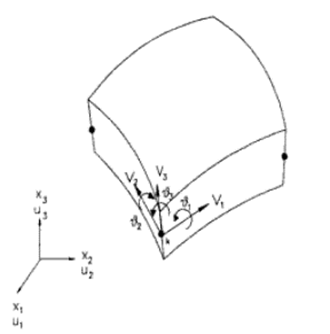A generic point of a shell may be described in terms of the position vectors of the nodes and shape functions. Two dimensional shape functions are used to describe a position in the element. Nk is the two dimensional shape function in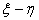plane. Hk is the one dimensional shape function along the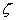axis.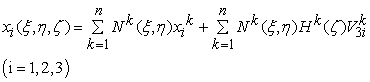(1)

Where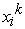is the position vector of node k in the reference surface;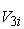is the unit vector at the node k; and n is the number of nodes per element.

The unit vector is defines as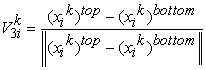(2)

Where top and bottom indicate the top and bottom surfaces of the shell and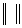denotes the euclidean norm. The one dimensional shape function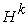is expressed as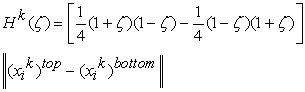(3)

In whichindicates the location of the reference surface and varies from -1 to +1.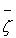=0 represents the mid surface. The shape functions are obtained as follows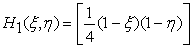(4)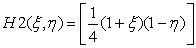(5)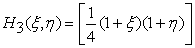(6)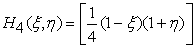(7)

The displacement field in a shell can be written as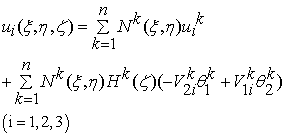(8)

In which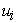is the displacement along the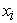axis,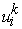is the nodal displacement at the node k and unit vectors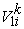,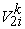lie along the reference surface.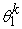,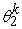are rotational degrees of freedom along the unit vectors.

3.2. Strain Displacement Relations

Six strain components are computed from above equation by taking the derivative with respect to the xi axis. The matrix takes the form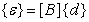(9)

and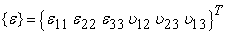(10)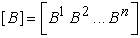(11)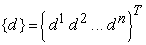(12)

The expression [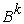] is given by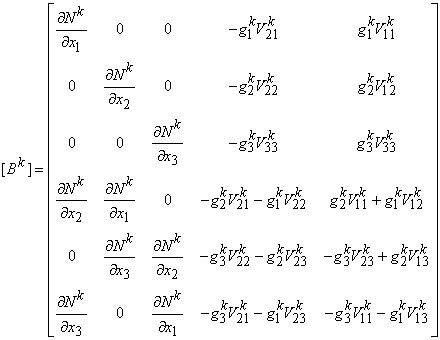(13)

In which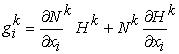(14)

In addition the vector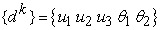(15)

Constitutive equations

Stresses and strains can be expressed as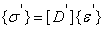(16)

Where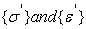are the stresses and strain components in the local axes along the reference plane. The constitutive matrix is given as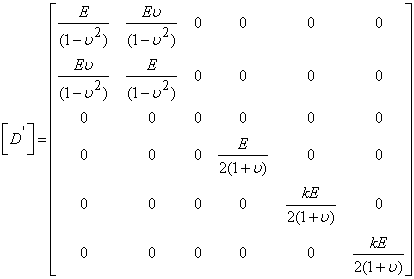(17)

In which E and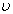are the elastic and poisons ratio respectively. The material property matrix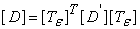(18)

Where the transformation matrix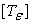is (19)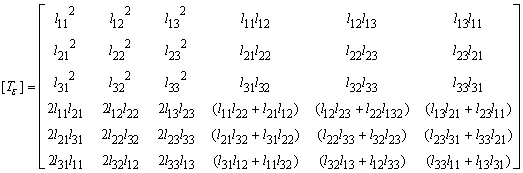(19)

Where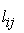are the directional cosines of the unit vector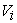with respect to the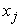axis

Element stiffness matrix

The element stiffness matrix is computed from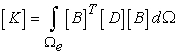(20)

And the element mass matrix is given as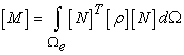(21)

In general modal analysis consists of extraction of modal parameters i.e. Eigen frequencies; mode shapes of modal damping thesis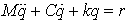(22)

Where M, C, K mass, damping, stiffness matrix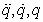acceleration, velocity, displacement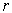external force vector

The equation (22) Compose the governing discrete motion, time invariant mathematical basis for modal analysis. However, the specific application of (22) differs in the type of analysis. In this work general modal analysis type in the form of free vibration are considered.

3.3. Parametric Extraction of Free Response System

The numerical modal parameter will be extracted from finite element response analysis. For curved blade, neglecting the effect of damping in the finite element analysis, the free response analysis are described by the homogeneous equation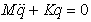(23)

The natural frequency are obtained using (23) and corresponding mode shapes are plotted by using the natural frequencies.

### 4. Results &Conclusions

In this present study the effect of pre twist, fiber orientations and layer lamination on the vibration response of a twisted blade are considered. To investigate the difference in vibration response for the blade shell 181 elements are used for finite element modeling and the results are varied in steps of twist angles from 0 to 90. The table shows the quantative comparisons of the 10 natural frequencies of a non-rotating blade. The blade is meshed up with shell elements using Hyper mesh solver and the numerical results are obtained using ANSYS. Table 4 shows the non-dimensional frequency parameters of twisted composite blade model. The Table 3 shows the natural frequencies of a Titanium blade with various pre twist angles and the corresponding graphs represents the mode shapes as a function of natural frequencies and pre twist angles. The Table 4 shows the variation in natural frequencies of a pre twisted blade at an angle of 18. Table 5 shows the results of composite blades with various pre twist angles.

### 5. Conclusions

In this study vibration characteristics of a composite curved blade were analyzed using the finite element approach. The blade was modeled as a cantilever oriented arbitrarily with respect to the rotating axes. The effects of initial twist, fiber orientation, and composite laminates were investigated on the vibration characteristics of composite blade. Results of the analysis of the present study can be summarized as follows:

From Table 3, Table 5 for an initially twisted non rotating blade the bending frequency tend to decrease whereas the twisting frequency tends to increase with the increase in the initial twist.

From Table 4 it can observed that with increase in number of layers from 6 to 50 with symmetric ply sequence the operating frequencies are increased by 6%.

The different plyups changed the natural frequencies of the composite blades with this change the desired vibrations characteristics of the blade without modifying the shape is expected. Therefore initial twist and ply lays should be optimized when rotating blades are designed to obtain maximum fundamental natural frequencies

### References

  Hung HeeYoo, Jung Hun Park, Jagh Yun Park: vibration analysis of rotating pre twisted blades, Computers & Structures 79 (2001) 1811-1819.In article CrossRef  Carnegie W: Vibration of Pretwisted Cantilever blading allowing for rotating inertia and Shear deflection, Journal of Mechanics Engineering & Science 1964: 105.In article CrossRef  Dunholter, R.J: Static displacement and coupled frequencies of a twisted bar, J.Aero. sci. 13, p. 214 (1946).In article  Subramanyam K.B. & Rao J.S. Coupled Bending Vibrations of Pretwisted Tapered Cantilever beams treated by Reissner method, Journal of Sound &Vibration (1982) 82 (4), 577-598.In article CrossRef  South well R, Gough F: The free transverse vibration of airscrewblades. British A.R.C. Reports& Memoranda no. 766, 1921.In article  MABIE. H. H & ROGERS C. B: Transverse Vibrations of Doublee-Tapered Cantilever Beams.In article  Carneige.W: Vibrations of pretwisted cantilever blading, Proceedings of the Institution of Mechanical engineers 1959 173: 343.In article CrossRef  White, W.T: An integral equation approach to problems of vibrating beams Franklin Ins., 245, pp. 25 and 117(1948)In article  Diprima, R.C. and Handle man, G.H: Vibration of twisted beams, Q. appl. Math. 12, p. 241 (1954).In article  Martin, A.I: approximation for the effect of twist on vibration of cantilever blade, Aero. p. 291 (1957).In article  Rao, J.S: Flexural vibrations of pre twisted tapered cantilever beams treated by Galerkin method, J.eng. Indus. ASME, 94, p. 343 (1972).In article  Sheng-An Liu, Chen-Yee Hung, Chun-Ying Lee: Vibration analysis of Pretwisted rotating composite wind mill blade by FEM.In article  Toshio Yamatogi, Hideaki Murayama, Kiyoshi Uzawa, Takahiro Mishima, Yasuaki Ishihara: Study on Composite Material Marine Propellers, Journal of the JIME Vol. 46. No. 3 ©JIME 2004.In article  Mohammad Nabi S & Ganeshan N: Comparison of Beam and Plate theories for free vibration of metal matrix composite pretwisted blades, Journal of Sound and Vibration (1996) 189(2), 149-160.In article CrossRef  Sahu.S.K., Asha.A.V.& Mishra R.N: Stability of laminated composite pretwisted cantilever panels, Journal of Reinforced and composites, 2005, 24 (12),1327-1334.In article  J.G.Russel: Use of reinforced plastics in a composite propeller blade, plastics and polymers, Dec 1973, pp 292-296.In article  Bhumbla R, Kosmatka JB, Reddy JN: Free vibration behavior of spinning shear-deformable plates composed of composite materials. AIAA J 1990; 28 (11): 1962-70.In article CrossRef  M. K. Pandit, S. Haldar, M. Mukhopadhyay, Free Vibration Analysis of Laminated Composite Rectangular Plate Using Finite Element Method, Journal of Reinforced Plastics and Composites January 2007 vol. 26 no. 1 69-80.In article CrossRef  Ihsan Küçükrendeci and Ömer K. Morgül Effect of elastic boundary conditions on free vibration of laminated composite plates, Scientific Research and Essays Vol. 6 (19), pp. 3949-3958, 8 September, 2011, Available online at http://www.academicjournals.org/SREIn article  Ngo Nhu Khoa. and Tran Ich Thinh. 2007. Finite Element Analysis of Laminated Composite Plates Using High Order Shear Deformation Theory. “Vietnam Journal of Mechanics”, VAST, Vol. 29, No. 1, pp. 47-57.In article  B. P. Patel, M. Ganapathi and S. Kamat, “Free vibration characteristics of laminated composite joined conical-cylindrical shells, Journal of Sound and vibration, Vol. 237, No. 5, 2000, pp. 920-930.In article